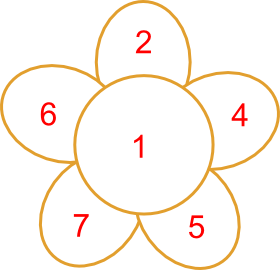#### You may also like### Consecutive Numbers

An investigation involving adding and subtracting sets of consecutive numbers. Lots to find out, lots to explore.### Calendar Capers

Choose any three by three square of dates on a calendar page...Make a set of numbers that use all the digits from 1 to 9, once and once only. Add them up. The result is divisible by 9. Add each of the digits in the new number. What is their sum? Now try some other possibilities for yourself!

# Number Daisy

##### Age 11 to 14Challenge Level
 1 = 1 2 = 2 3 = 1 + 2 4 = 4 5 = 5 6 = 2 + 4 7 = 1 + 2 + 4 8 = 5 + 1 + 2 9 = 4 + 5 10 = 7 + 1 + 2 11 = 5 + 4 + 2 12 = 5 + 4 + 2 + 1 13 = 7 + 6 14 = 7 + 6 + 1 15 = 7 + 6 + 2 16 = 7 + 6 + 2 + 1 17 = 7 + 5 + 4 + 1 18 = 7 + 5 + 4 + 2 19 = 7 + 5 + 4 + 2 + 1 20 = 5 + 7 + 6 + 2 21 = 5 + 7 + 6 + 2 + 1 22 = 4 + 5 + 7 + 6 23 = 5 + 7 + 6 + 1 + 4 24 = 5 + 7 + 6 + 2 + 4 25 = 5 + 7 + 6 + 2 + 4 + 1 This Daisy is special because you can make every number from 1 to 25. You are only allowed to add neighbours (numbers touching each other) and you can only use each number once in a sum.We can make all the numbers from 1 to 25 using this Daisy.
Can you do better than this with a different set of numbers?
The challenge is to find six numbers to go in the Daisy from which you can make all the numbers from 1 to a number bigger than 25.

This task was found in one of Brian Bolt's books and developed by MEDIAN in their collection of interesting number resources.